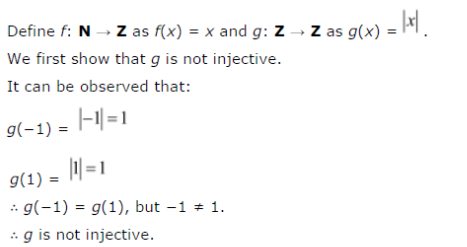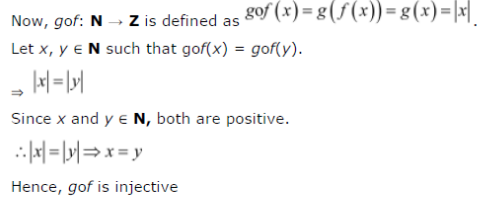# Give examples of two functions f : N → N and g : Z → Z such that

Give examples of two functions f : N → N and g : Z → Z such that gof is injective but g is not injective.
(Hint: Consider f(x) = x and g(x) = |x|)